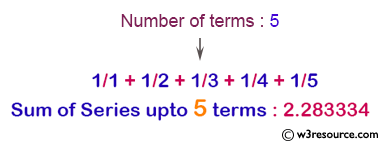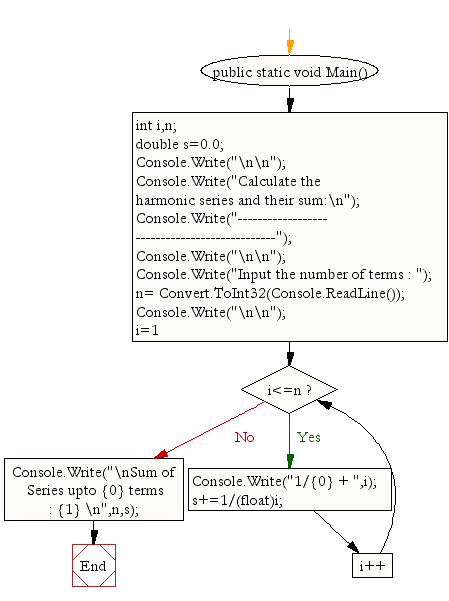﻿ C# - Calculate the harmonic series and their sum# C# Sharp Exercises: Calculate the harmonic series and their sum

## C# Sharp For Loop: Exercise-19 with Solution

Write a program in C# Sharp to display the n terms of harmonic series and their sum. The series is :
1 + 1/2 + 1/3 + 1/4 + 1/5 ... 1/n terms

Pictorial Presentation:Sample Solution:

C# Sharp Code:

``````using System;
public class Exercise19
{
public static void Main()
{
int i,n;
double s=0.0;

Console.Write("\n\n");
Console.Write("Calculate the harmonic series and their sum:\n");
Console.Write("----------------------------------------------");
Console.Write("\n\n");

Console.Write("Input the number of terms : ");
Console.Write("\n\n");
for(i=1;i<=n;i++)
{
Console.Write("1/{0} + ",i);
s+=1/(float)i;
}
Console.Write("\nSum of Series upto {0} terms : {1} \n",n,s);
}
}
```
```

Sample Output:

```
Calculate the harmonic series and their sum:
----------------------------------------------
Input the number of terms : 6
1/1 + 1/2 + 1/3 + 1/4 + 1/5 + 1/6 +
Sum of Series upto 6 terms : 2.45
```

Flowchart:C# Sharp Code Editor:

Contribute your code and comments through Disqus.

What is the difficulty level of this exercise?

Test your Programming skills with w3resource's quiz.

﻿